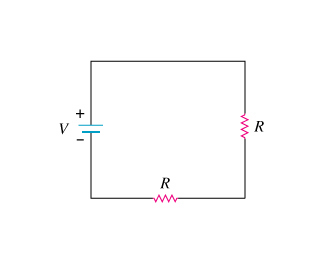# What is the power, in terms of Po, dissipated by this circuit?

edited

What is the power, in terms of Po, dissipated by this circuit?

A single resistor is wired to a battery as shown in the diagram below. Define the total power dissipated by this circuit as Po.

Figure 1:Now, a second identical resistor is wired in series with the first resistor as shown in the second diagram to the left.

Figure 2:What is the power, in terms of Po, dissipated by this circuit?

by

Figure 1 P_o_1 = V^2/R

Figure 2 P_o_2 = V^2/[2R]

So P_o_1 = 2 * P_o_2

Essentially, resistance has doubled and the voltage has stayed the same, so current and power will be halved.

Lorem ipsum dolor sit amet, consectetur adipiscing elit, sed do eiusmod tempor incididunt ut labore et dolore magna aliqua. Ut enim ad minim veniam, quis nostrud exercitation ullamco laboris nisi ut aliquip ex ea commodo consequat. Duis aute irure dolor in reprehenderit in voluptate velit esse cillum dolore eu fugiat.

+1 vote